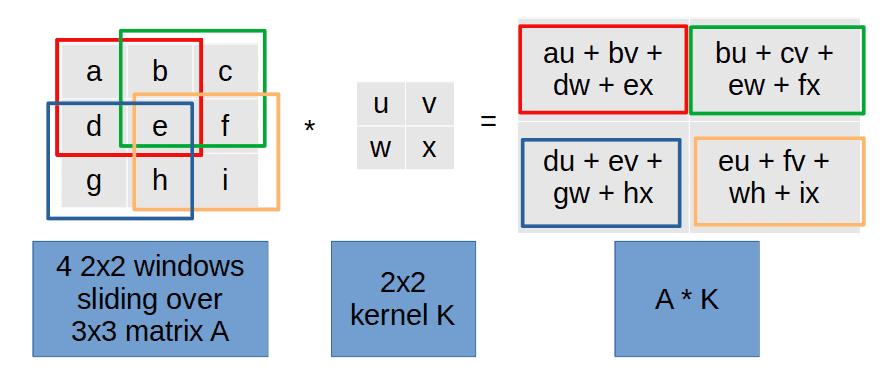## 1. Introduction

In this tutorial, we’ll show a matrix approach to do 2D convolution.

## 2. Convolution

Convolution is fundamental in signal processing, computer vision, and machine learning. It applies a filter or kernel to an input image or signal and extracts relevant features.

Typical implementations use a sliding-window operation where the kernel moves across the input image. For each placement of the kernelon the input image, we compute the dot product of the kernel and the overlapping image pixels:However, the kernel approach can be computationally expensive, especially for large images and kernels. An alternative is to compute convolution as matrix multiplication, which can be a more efficient approach.

## 3. Matrix Construction of a 2D Convolution

Letbe a matrix of sizeandakernel. For convenience, letTo calculate the convolution, we need to calculate the matrix-vector multiplicationwhere:

•is ablock matrix we get from the kernel•is a row vector with theelements ofconcatenated row by row
•is a row vector ofelements which can be reshaped into amatrix by splitting the row vector intorows ofelements

### 3.1. How to Compute the Block Matrix?

It’s a three-step process.

First, we define thematrixes, which represent all the horizontal sliding windows for kernel row:witha zero row vector of sizeandthe-th element of row, i.e. the element at rowand columnin matrix.stands for an empty vector.

In the second step, we complete horizontal sliding by constructing amatrix. We defineas the horizontal concatenation of matrix blocks:The final step is to represent vertical window sliding. We do that by paddingwith zero matrices (denoted):All that is now left to do is compute, whereis a row-vector form of the input matrix.

## 4. Example

Let’s construct the matrixfor amatrixconvolved with akernel:In this example, we have. First, we build the blocksand:and getafter concatenation:The final step is to pad the matrix with blocks of zeros:The zeros in thematrix blocks are highlighted in red, andis exactly of the size.

Now, let’s rewriteas:Finally, we multiplyand:Since, we obtain the result of the convolutionas amatrix by simplifying and reshaping:### 4.1. Numeric Example

Let’s sayandare:The corresponding,, andare as follows:The result of the convolution is:We can reshape:## 5. Advantages of the Matrix Approach

Matrix multiplication is easier to compute compared to a 2D convolution because it can be efficiently implemented using hardware-accelerated linear algebra libraries, such as BLAS (Basic Linear Algebra Subprograms). These libraries have been optimized for many years to achieve high performance on a variety of hardware platforms.

In addition, the memory access patterns for matrix multiplication are more regular and predictable compared to 2D convolution, which can lead to better cache utilization and fewer memory stalls. This can result in faster execution times and better performance.

## 6. Conclusion

In this article, we showed how to compute a convolution as a matrix-vector multiplication. The approach can be faster than the usual one with sliding since matrix operations have fast implementations on modern computers.